### Example 3.13 Balanced Incomplete Block Design

Balanced incomplete block design (BIBD) generation is a standard combinatorial problem from design theory. The concept was originally developed in the design of statistical experiments; applications have expanded to other fields, such as coding theory, network reliability, and cryptography. A BIBD is an arrangement ofdistinct objects intoblocks such that each block contains exactlydistinct objects, each object occurs in exactlydifferent blocks, and every two distinct objects occur together in exactlyblocks. A BIBD is therefore specified by its parameters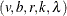. It can be proved that when a BIBD exists, its parameters must satisfy the conditions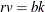,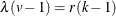, and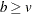, but these conditions are not sufficient to guarantee the existence of a BIBD (Prestwich, 2001). For instance, the parameters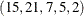satisfy the preceding conditions, but a BIBD with these parameters does not exist. Computational methods for BIBD generation generally suffer from combinatorial explosion, in part because of the large number of symmetries: given any solution, any two objects or blocks can be exchanged to obtain another solution.

This example demonstrates how to express a BIBD problem as a CSP and how to use lexicographic ordering constraints to break symmetries. The most direct CSP model for BIBD, as described in Meseguer and Torras (2001), represents a BIBD as a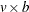matrix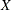. Each matrix entry is a Boolean decision variable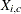that satisfies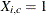if and only if blockcontains object. The condition that each object occurs in exactlyblocks (or, equivalently, that there are1s per row) can be expressed aslinear constraints: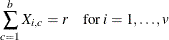Alternatively, you can use global cardinality constraints to ensure that there are exactly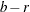0s and1s in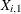,…,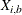for each object: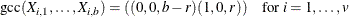Similarly, the condition that each block contains exactlyobjects (there are1s per column) can be specified by the following constraints: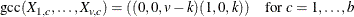To enforce the final condition that every two distinct objects occur together in exactlyblocks (equivalently, that the scalar product of every pair of rows is equal to), you can introduce auxiliary variables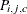for every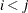that indicate whether objectsandboth occur in block. The REIFY constraint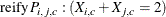ensures that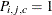if and only if blockcontains both objectsand. The following constraints ensure that the final condition holds: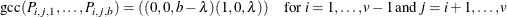The objects and the blocks are interchangeable, so the matrixhas total row symmetry and total column symmetry. Because of the constraints on the rows, no pair of rows can be equal unless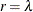. To break the row symmetry, you can impose strict lexicographical ordering on the rows ofas follows: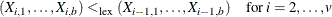To break the column symmetry, you can impose lexicographical ordering on the columns ofas follows: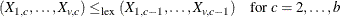The following SAS macro incorporates all the preceding constraints. For specified parameters, the macro either finds BIBDs or proves that a BIBD does not exist.

%macro bibd(v, b, r, k, lambda, out=bibdout);
/* Arrange v objects into b blocks such that:
(i) each object occurs in exactly r blocks,
(ii) each block contains exactly k objects,
(iii) every pair of objects occur together in exactly lambda blocks.

Equivalently, create a binary matrix with v rows and b columns,
with r 1s per row, k 1s per column,
and scalar product lambda between any pair of distinct rows.
*/

/* Check necessary conditions */
%if (%eval(&r * &v) ne %eval(&b * &k)) or
(%eval(&lambda * (&v - 1)) ne %eval(&r * (&k - 1))) or
(&v > &b) %then %do;
%put BIBD necessary conditions are not met.;
%goto EXIT;
%end;

proc clp out=&out(keep=x:) domain=[0,1] varselect=FIFO;
/* Decision variables: */
/* Decision variable X_i_c = 1 iff object i occurs in block c. */
var (
%do i=1 %to &v;
x&i._1-x&i._&b.
%end;
) = [0,1];

/* Mandatory constraints: */
/* (i) Each object occurs in exactly r blocks. */
%let q = %eval(&b.-&r.);  /* each row has &q 0s and &r 1s */
%do i=1 %to &v;
gcc( x&i._1-x&i._&b. ) = ((0,0,&q.) (1,0,&r.));
%end;

/* (ii) Each block contains exactly k objects. */
%let h = %eval(&v.-&k.);  /* each column has &h 0s and &k 1s */
%do c=1 %to &b;
gcc(
%do i=1 %to &v;
x&i._&c.
%end;
) = ((0,0,&h.) (1,0,&k.));
%end;

/* (iii) Every pair of objects occurs in exactly lambda blocks. */
%let t = %eval(&b.-&lambda.);
%do i=1 %to %eval(&v.-1);
%do j=%eval(&i.+1) %to &v;
/* auxiliary variable p_i_j_c =1 iff both i and j occur in c */
var ( p&i._&j._1-p&i._&j._&b. ) = [0,1];
%do c=1 %to &b;
reify p&i._&j._&c.: (x&i._&c. + x&j._&c. = 2);
%end;

gcc(p&i._&j._1-p&i._&j._&b.) = ((0,0,&t.) (1,0,&lambda.));
%end;
%end;

/* Symmetry breaking constraints: */
/* Break row symmetry via lexicographic ordering constraints. */
%do i = 2 %to &v.;
%let i1 = %eval(&i.-1);
lexico( (x&i._1-x&i._&b.) LEX_LT (x&i1._1-x&i1._&b.) );
%end;

/* Break column symmetry via lexicographic ordering constraints. */
%do c = 2 %to &b.;
%let c1 = %eval(&c.-1);
lexico( ( %do i = 1 %to &v.;
x&i._&c.
%end; )
LEX_LE
( %do i = 1 %to &v.;
x&i._&c1.
%end; ) );
%end;
run;
%put &_orclp_;
%EXIT:
%mend bibd;


The following statement invokes the macro to find a BIBD design for the parameters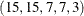:


%bibd(15,15,7,7,3);



The output is displayed in Output 3.13.1.

Output 3.13.1: Balanced Incomplete Block Design for (15,15,7,7,3)

 Balanced Incomplete Block Design Problem (15, 15, 7, 7, 3)

Obs Block1 Block2 Block3 Block4 Block5 Block6 Block7 Block8 Block9 Block10 Block11 Block12 Block13 Block14 Block15
1 1 1 1 1 1 1 1 0 0 0 0 0 0 0 0
2 1 1 1 0 0 0 0 1 1 1 1 0 0 0 0
3 1 1 0 1 0 0 0 1 0 0 0 1 1 1 0
4 1 0 1 0 1 0 0 0 1 0 0 1 1 0 1
5 1 0 0 1 0 1 0 0 0 1 1 1 0 0 1
6 1 0 0 0 1 0 1 0 0 1 1 0 1 1 0
7 1 0 0 0 0 1 1 1 1 0 0 0 0 1 1
8 0 1 1 0 0 0 1 0 0 1 0 1 0 1 1
9 0 1 0 1 0 0 1 0 1 0 1 0 1 0 1
10 0 1 0 0 1 1 0 1 0 1 0 0 1 0 1
11 0 1 0 0 1 1 0 0 1 0 1 1 0 1 0
12 0 0 1 1 1 0 0 1 0 0 1 0 0 1 1
13 0 0 1 1 0 1 0 0 1 1 0 0 1 1 0
14 0 0 1 0 0 1 1 1 0 0 1 1 1 0 0
15 0 0 0 1 1 0 1 1 1 1 0 1 0 0 0